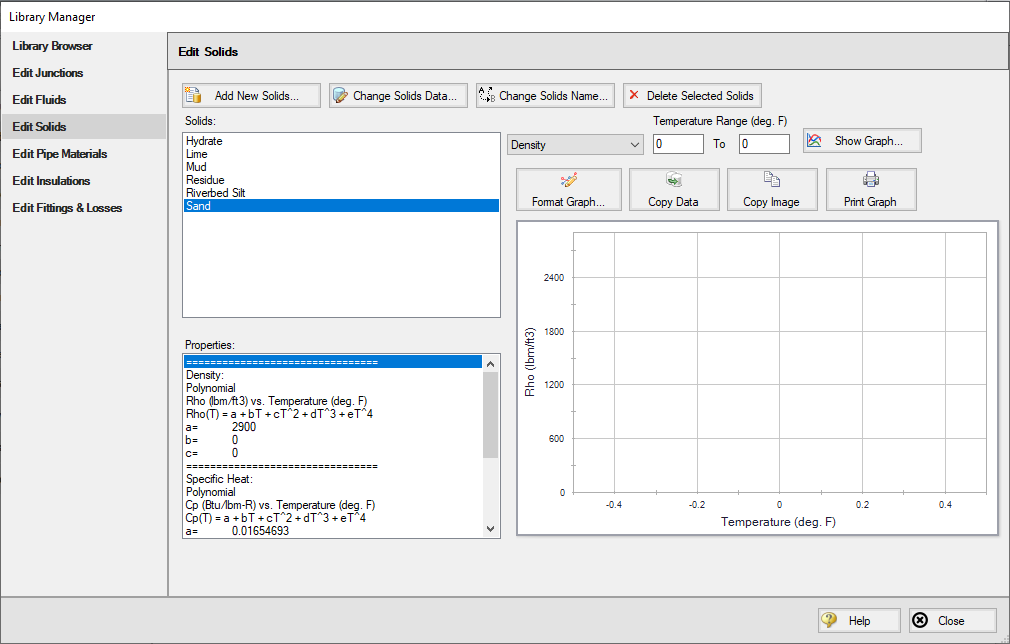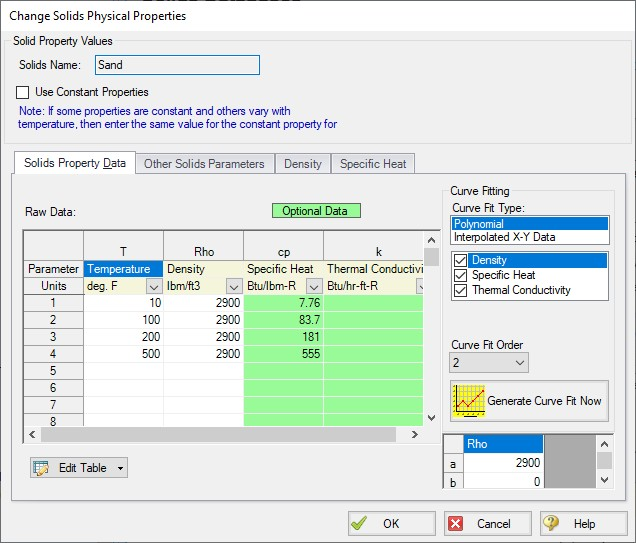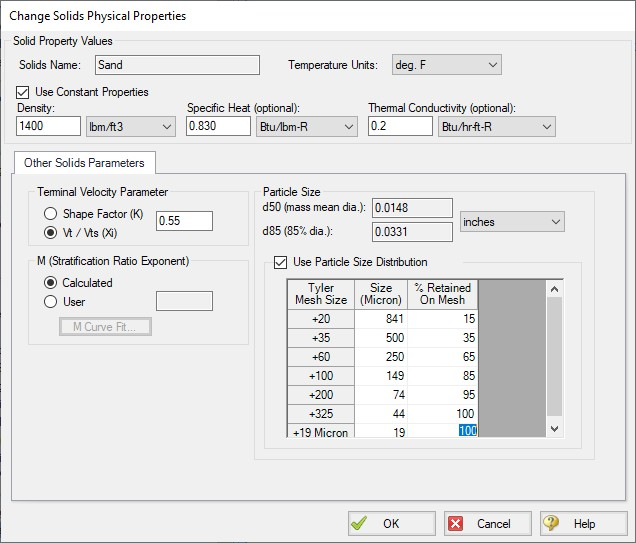### Solids Library

(SSL Module Only) Users can create Solids Library entries which retain data for specific solids profiles. The Solids Library window (Figure 1) can be accessed from the Library menu or from Analysis Setup on the Solids Definition panel.

This allows you to view, add, edit and delete solids in the library. The physical properties are entered in the Change Solids Physical Properties window. Enter the name of the solids data and the temperature units.

Often the physical properties of the solids do not vary appreciably with temperature or the variation is unknown. In this case select Use Constant Properties (Figure 1). The temperature units are ignored but one must still be selected. The density is always required. The specific heat and thermal conductivity are optional and are only used when heat transfer is modeled.

If one or more properties vary with temperature, uncheck the Use Constant Properties box. The property values as a function of temperature can then be entered in the table on the Solids Property Data tab (Figure 2). If a property does not vary with temperature, simply enter the same value for each temperature listed in the table.Figure 1: Solids Library showing example of user-entered solids dataFigure 2: Change Solids Physical Properties window showing tab to specify thermophysical properties of solids (available when “Use Constant Properties” is unchecked)

Other Solids Parameters

The Terminal Velocity Parameter and the M exponent are entered on the Other Solids Parameters tab (Figure 3). The particle size distribution is also entered on this tab.

Similar to direct entry on the Solids Definition panel, the user can allow AFT Fathom to estimate the M factor from recorded data or can input an M value directly. If M is calculated values for both d50 and d85 must be entered.

The Terminal Velocity Parameter can be entered as either a Shape Factor (K) or the ratio of the particle's terminal velocity (Vt) to that of a sphere (Vts). This ratio is designated by z or Xi

Typical shape factors are given in Wilson, et al, 2006, on page 46 where K = 0.26 for sand. To ignore this effect select Vt / Vts = 1.

Particle Size Distribution

The particle size parameters d50 and d85 can be entered directly or statistically calculated based on particle size distribution data.

To have Fathom calculate d50 and d85 check the Use Particle Size Distribution option, then enter the percent of the solids that remains on the mesh as observed from test data for each Tyler Mesh Size. The d50 and d85 parameters will automatically be calculated (Figure 3).Figure 2: Solids Library showing Solids Parameters allows definition of particle size distribution

Related Topics